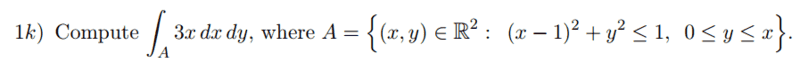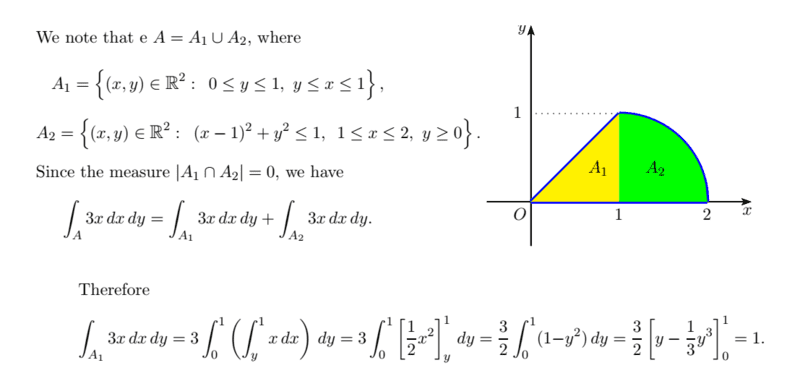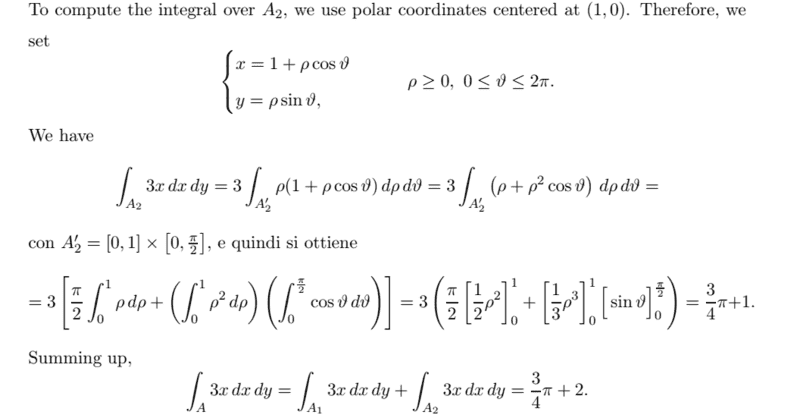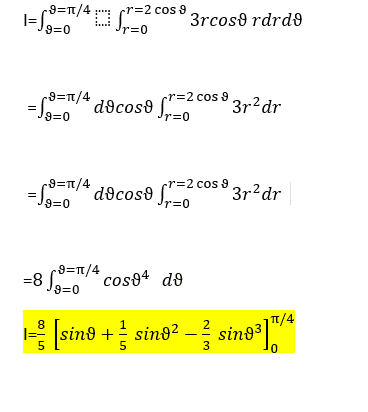# Double integral with polar coordinates

Homework Statement:
look at the image
Relevant Equations:
polar coordinates
Greetings!
I have the following integraland here is the solution of the book (which I understand perfectly)I have an altenative method I want to apply that does not seems to gives me the final result

My methodwhich doesn't give me the final results!
where is my mistake?
thank you!

ergospherical
Gold Member
You can write \begin{align*}
I &= \int_0^{\pi/4} \int_0^{\sqrt{2+2\cos{2\theta}}} 3r^2 dr d\theta \\
&= \int_0^{\pi/4} \cos{\theta} \left( 2+2\cos{2\theta}\right)^{3/2} d\theta \\
&= 8 \int_0^{\pi/4} \cos^4{\theta} d\theta
\end{align*}then\begin{align*}
\int \cos^4{x} dx &=\int \dfrac{(e^{ix} + e^{-ix})^4}{16} dx \\
&= \int \left(\dfrac{e^{4ix} + e^{-4ix} + 4e^{2ix} + 4e^{-2ix} + 6}{16} \right) dx \\
&= \int \left( \dfrac{1}{8} \cos{4x} + \dfrac{1}{2} \cos{2x} + \dfrac{3}{8} \right) dx \\
&= \dfrac{1}{32} \sin{4x} + \dfrac{1}{4} \sin{2x} + \dfrac{3}{8} x \bigg{|}_{0}^{\pi/4} \\
&= \dfrac{1}{4} + \dfrac{3\pi}{32}
\end{align*}

Last edited:
You can write
\begin{align*}
I &= \int_0^{\pi/4} \int_0^{\sqrt{2+2\cos{2\theta}}} 3r^2 dr d\theta \\
&= \int_0^{\pi/4} \cos{\theta} \left( 2+2\cos{2\theta}\right)^{3/2} d\theta \\
&= 8 \int_0^{\pi/4} \cos^4{\theta} d\theta
\end{align*}
then
\begin{align*}
\int \cos^4{x} dx &=\int \dfrac{(e^{ix} + e^{-ix})^4}{16} dx \\
&= \int \left(\dfrac{e^{4ix} + e^{-4ix} + 4e^{2ix} + 4e^{-2ix} + 6}{16} \right) dx \\
&= \int \left( \dfrac{1}{8} \cos{4x} + \dfrac{1}{2} \cos{2x} + \dfrac{3}{8} \right) dx \\
&= \dfrac{1}{32} \sin{4x} + \dfrac{1}{4} \sin{2x} + \dfrac{3}{8} x \bigg{|}_{0}^{\pi/4} \\
&= \dfrac{1}{4} + \dfrac{3\pi}{32}
\end{align*}

ergospherical
Gold Member
Yeah I don't know why that's happening, the LaTeX is fine and works when I run it on Overleaf.•Amaelle
@ergospherical thank you very much!
indeed my approach was correct, I only messed up with the calculation when I was trying to integrate 8*cos^5 instead of 8*cos^4
thank you again!

berkeman
Mentor
Yeah I don't know why that's happening, the LaTeX is fine and works when I run it on Overleaf
It looks like you had no space before \begin and left off the ## delimiters at start/end. I fixed it but you deleted it as I was fixing it...

•SammyS